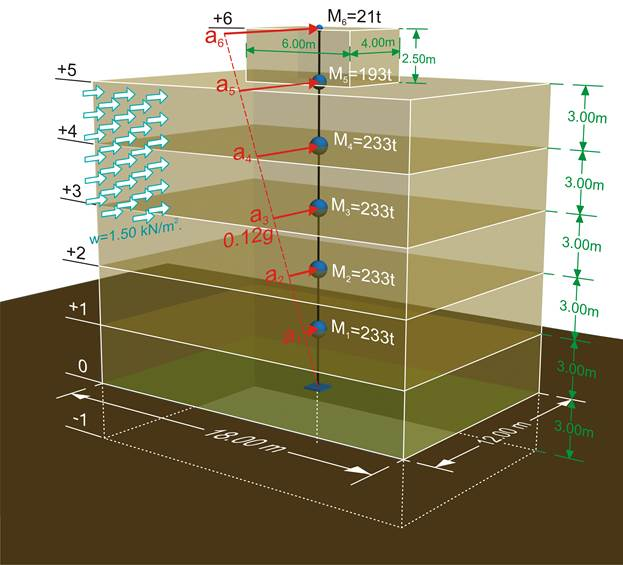# Wind and Seismic Forces >Introduction >
The structural frame
The construction
The reinforcement I
The reinforcement II
Quantity/Cost estimation
Detailing drawingsIntroduction >
Wind and Seismic Forces >
Structural model and Analysis
Modelling slabs
Slabs
Seismic behavour of frames
Appendix A
Appendix B
Appendix C
Appendix DIntroduction >
Materials
To be continued >

## Comparison of wind and seismic forces ExampleThe geometrical and loading model of the building's wind and seismic forces

The residential building of the sketch includes a basement of area 12×18 m2 and height 3 m, a ground floor and four storeys of identical dimensions and a top floor of area 4×6 m2 and height 2.5 m. The masses at levels 0, 1, 2, 3, 4 are equal to MG=220 t and MQ=44 t, at level 5 to MG=180 t and MQ=44 t, while at the top level to MG=20 t and MQ=4 t. The building is situated in the seismic area Z1 and the distribution of seismic accelerations is triangular. The design seismic acceleration of magnitude 0.12g is applied at the center of mass of the building.

## Wind and seismic forcesWind forces Fw are less significant comparing to earthquake forces Fs

Wind forces represent 388/1349=29% of the seismic forces and their CM is at (1/2)/(2/3)=75% of the CM of seismic forces. Consequently the seismic forces are of much greater value as well as importance than the wind forces.